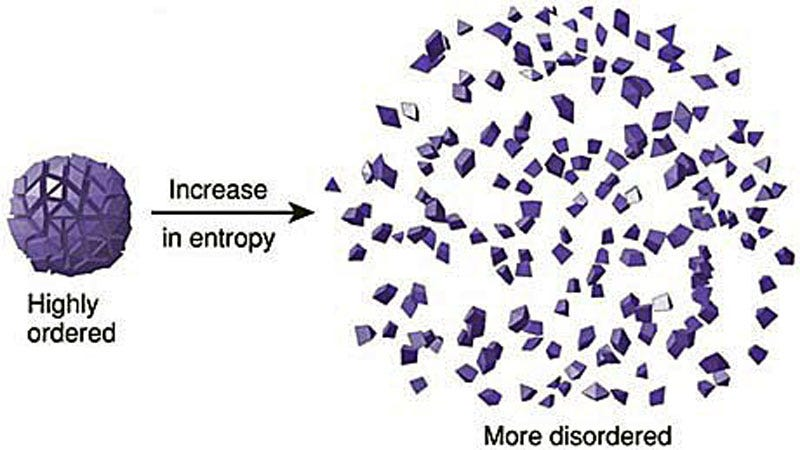# Maximum Entropy Reinforcement Learning

Original article was published by Dhanoop Karunakaran on Artificial Intelligence on Medium# Maximum Entropy Reinforcement Learning

Let’s discuss entropy before diving into the usage of entropy in Reinforcement Learning(RL).

# Entropy

Entropy is an old concept in physics. It can be defined as the measure of chaos or disorder in a system. Higher entropy means lower chaos. It is slightly different in information theory. The mathematician Claude Shannon introduced the entropy in information theory in 1948. Entropy in information theory can be defined as the expected number of bits of information contained in an event. For instance, tossing a fair coin has the entropy of 1. It is because of the probability of having a head or tail is 0.5. The amount of information required to identify it’s head or tail is one by asking one, yes or no question — “is it head ? or is it tail?”. If the entropy is higher, that means we need more information to represent an event. Now, we can say that entropy increases with increases in uncertainty. Another example is that crossing the street has less number of information required to represent/ store/ communicate than playing a poker game.

Calculating how much information is in a random variable, X={x0,x1,….,xn} is same as calculating the information for the probability distribution of the events in the random variable. In that sense, entropy is considered as average bits of information required to represent an event drawn from the probability distribution. Entropy for a random variable X can be computed using the below equation:

# Maximum Entropy Reinforcement Learning

In Maximum Entropy RL, the agent tries to optimise the policy to choose the right action that can receive the highest sum of reward and long term sum of entropy. This enables the agent to explore more and avoid converging to local optima. It is important to state the principle of maximum entropy to understand it uses in RL.

Principle of maximum entropy:

If we have a few number probability distribution that would encode the prior data, then the best probability distribution is the one with maximum entropy.

In the intuition of principle of maximum entropy, the aim is to find the distribution that has maximum entropy. In many RL algorithms, an agent may converge to local optima. By adding the maximum entropy to the objective function, it enables the agent to search for the distribution that has maximum entropy. As we defined earlier, in Maximum Entropy RL, the aim is to learn the optimal policy that can achieve the highest cumulative reward and maximum entropy. As the system has to search for the entropy as well, it enables more exploration and chances to avoid converging to local optima is higher.

The concept of adding entropy is not a new concept as there are RL algorithms that make use of entropy in the form of entropy bonus . Sometimes entropy is called entropy regularisation. For instance, entropy bonus in A3C RL algorithm. The entropy bonus is described as one step bonus as it focuses on the current state only and not much worry about the future states. As in standard RL, the aim of the agent to learn the optimal policy that can maximise the cumulative reward or long term reward. Similarly, learning the sum of entropy or long term entropy instead of learning the entropy in a one-time step has more benefits. The benefits are in terms of more robust performance under the changes in the agent’s knowledge about the environment and environment itself.

The optimal policy of the standard RL is as shown below. The agent learns to achieve the optimal policy which can receive a high cumulative reward by choosing an action from a given state.

The optimal policy of the Maximum entropy RL as shown below. The optimal policy is the highest expectation of long term reward and long term entropy.

If you like my write up, follow me on Github, Linkedin, and/or Medium profile.